# A Partition Filter Network for Joint Entity and Relation Extraction

## PFN

PFN由LSTM改进而来, 有LSTM基础再理解会好一些.

### Problem Formulation

• NER的任务目标是在给定实体起始Token$w_i$ 和结束Token$w_j$ 的情况下, 抽取出标有类别的实体$e \in \mathcal{E}$ , $\left\langle w_{i}, e, w_{j}\right\rangle \in S$.
• RE的任务目标是在给定Subject的起始Token $w_i$ 和Object的起始Token$w_j$ 的情况下, 抽取出二者存在的关系$r \in \mathcal{R}$, 即$\left\langle w_{i}, r, w_{j}\right\rangle \in T$, $T$ 为三元组.

### Partition Filter Encoder

PFN的Ecndoer就是被改进过的LSTM, 作者在LSTM基础上增加了PartitionFilter机制, 使得两任务之间的信息能够均衡合理的传递.

#### Partition

NER和RE之间存在很强的关联, 但每个任务也同样应该有自己独立的特征, 这部分独立特征是不该被另一个任务所影响的. 划分(Partition)机制的目标就是要学习到仅与本任务相关联的特征位置.

$$\tilde{c}_{t}=\tanh \left(\operatorname{Linear}\left(\left[x_{t} ; h_{t-1}\right]\right)\right)$$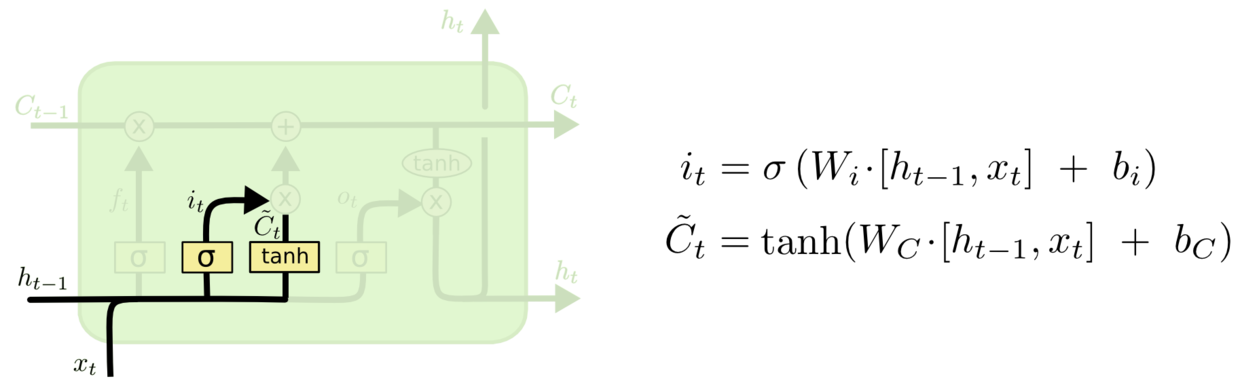$$\operatorname{cummax}(\cdot)=\operatorname{cumsum}(\operatorname{softmax}(\cdot))$$

$$\begin{gathered} \text { cumsum }\left(x_{1}, x_{2}, \ldots, x_{n-1}, x_{n}\right)=\left(x_{1}, x_{1}+x_{2}, \ldots,\right. \\ \left.x_{1}+x_{2}+\cdots+x_{n-1}, x_{1}+x_{2}+\cdots+x_{n-1}+x_{n}\right) \end{gathered}$$

$$\begin{gathered} \text { cumsum }\left(x_{1}, x_{2}, \ldots, x_{n-1}, x_{n}\right)=\left(\sum^1_{n=1}x_i, \sum^2_{n=1}x_i,\sum^{n-1}_{n=1}x_i,\cdots, \sum^n_{n=1}x_i\right) \end{gathered}$$
$\text{cummax}$ 的输出值可以近似成一个二进制向量$(0, \cdots, 0, 1, \cdots, 1)$. 举个栗子:
\begin{aligned} x &= (0.1, 0.1, 0.6, 0.1, 0.1) \\ \text{cumsum}(x) &= (0.1, 0.2, 0.8, 0.9, 1.0) \\ &\approx (0, 0, 1, 1, 1) \end{aligned}
NER和RE这两个任务都各有一个对输入划分的门控剪刀, 把输入分为任务相关, 任务不相关两块. 这两把剪刀分别记为$\tilde{e}$ 和$\tilde{r}$:
\begin{aligned} &\tilde{e}=\operatorname{cummax}\left(\operatorname{Linear}\left(\left[x_{t} ; h_{t-1}\right]\right)\right) \\ &\tilde{r}=1-\operatorname{cummax}\left(\operatorname{Linear}\left(\left[x_{t} ; h_{t-1}\right]\right)\right) \end{aligned}

\begin{aligned} \rho_{s, c_{t-1}} &=\tilde{e}_{c_{t-1}} \circ \tilde{r}_{c_{t-1}} \\ \rho_{e, c_{t-1}} &=\tilde{e}_{c_{t-1}}-\rho_{s, c_{t-1}} \\ \rho_{r, c_{t-1}} &=\tilde{r}_{c_{t-1}}-\rho_{s, c_{t-1}} \end{aligned}
$\tilde{e}, \tilde{r}$ 的结果做逐元素点乘, 就能获取共享部分, 细胞状态被切成了三份: NER区, RE区, Share区: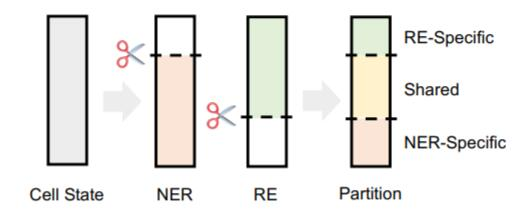\begin{aligned} \rho_{s, c_{t-1}} &= (0,1,1) \circ (1,1,0)=(0,1,0)\\ \rho_{e, c_{t-1}} &= (0,1,1)- (0,1,0)=(0,0,1)\\ \rho_{r, c_{t-1}} &= (1,1,0)- (0,1,0)=(1,0,0) \end{aligned}

\begin{aligned} \rho_{e} &=\rho_{e, c_{t-1}} \circ c_{t-1}+\rho_{e, \tilde{c}_{t}} \circ \tilde{c}_{t} \\ \rho_{r} &=\rho_{r, c_{t-1}} \circ c_{t-1}+\rho_{r, \tilde{c}_{t}} \circ \tilde{c}_{t} \\ \rho_{s} &=\rho_{s, c_{t-1}} \circ c_{t-1}+\rho_{s, \tilde{c}_{t}} \circ \tilde{c}_{t} \end{aligned}

$\rho$ 并不是PFN Encoder最终的细胞状态, 上述过程仅是与LSTM中的细胞状态更新相似: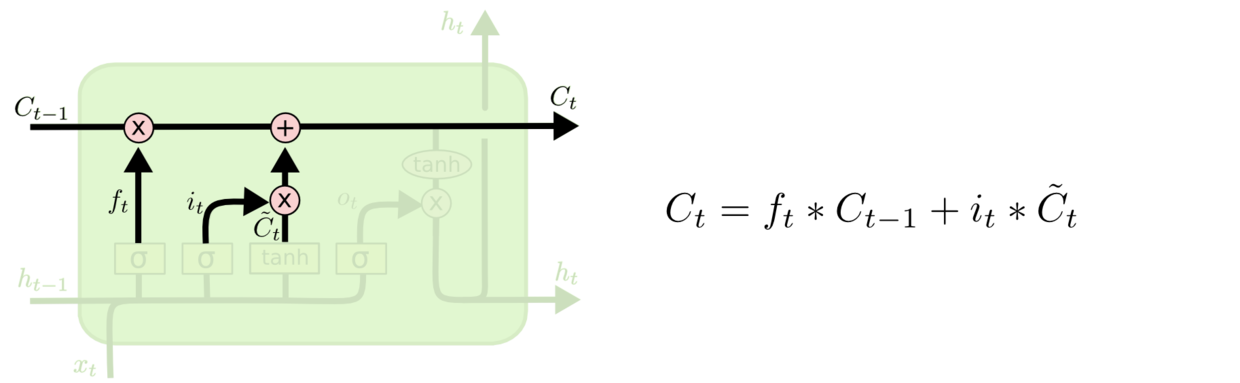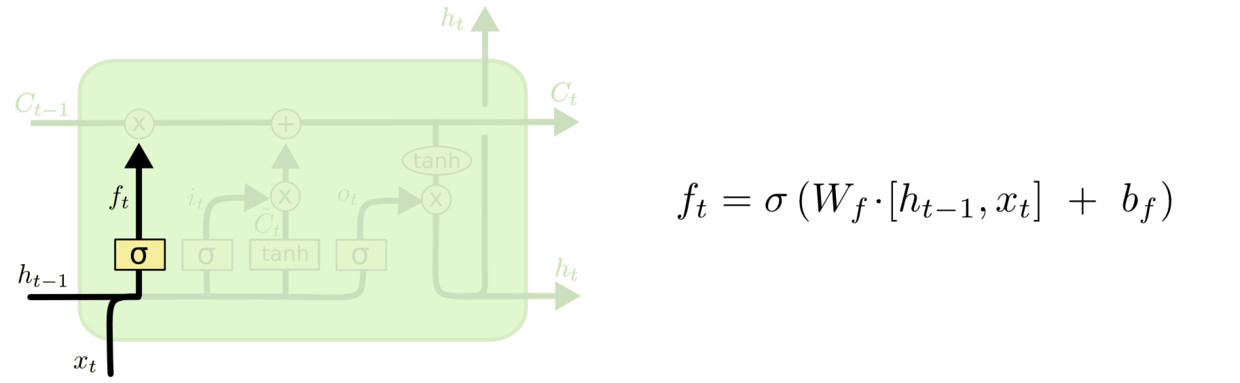#### Filter

NER可以使用独有的细胞状态$\rho_e$ 和共享细胞状态$\rho_s$, RE可以使用独有细胞状态$\rho_r$ 和共享细胞状态$\rho_s$, 对任务无关的信息直接被滤去:
\begin{aligned} \mu_{e}&=\rho_{e}+\rho_{s} \\\ \mu_{r}&=\rho_{r}+\rho_{s}\\ \mu_{s}&=\rho_{s} \end{aligned}

\begin{aligned} &h_{e}=\tanh \left(\mu_{e}\right) \\ &h_{r}=\tanh \left(\mu_{r}\right) \\ &h_{s}=\tanh \left(\mu_{s}\right) \end{aligned}

$h$ 将作为特定任务的表示, 在解码过程中使用.

\begin{aligned} c_{t} &=\operatorname{Linear}\left(\left[\mu_{e, t} ; \mu_{r, t} ; \mu_{s, t}\right]\right) \\ h_{t} &=\tanh \left(c_{t}\right) \end{aligned}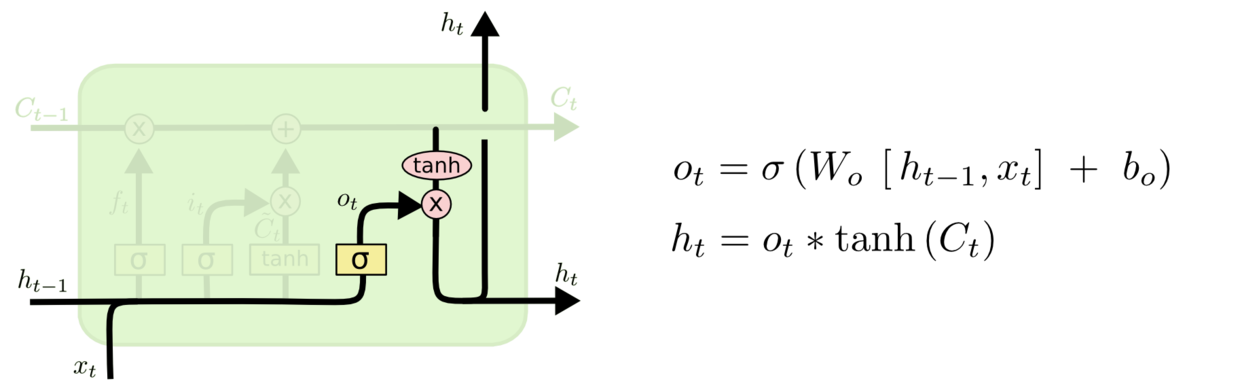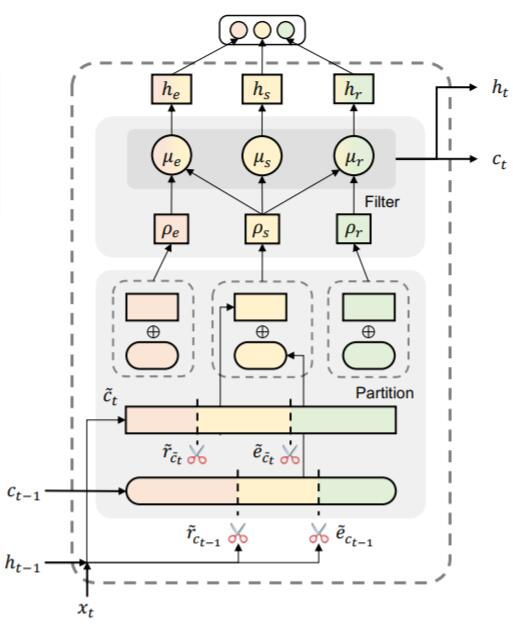1. 上时刻$t-1$ 被划分好的三份的细胞状态(NER区, RE区, Share区)记为$\rho_{c_{t-1}}$, 与当前时刻划分好的三份初始细胞状态$\rho_{\tilde{c}_t}$, 生成$t$ 时刻划分好的新细胞状态$\rho$.
2. 新细胞状态进一步过滤得到任务特化的细胞状态$\mu$.
3. 激活后得到任务所需的表征$h_e, h_r, h_s$.
4. 用类似输出门的方式得到当前时刻$t$ 的最终细胞状态$c_t$, 和输出隐态$h_t$.

### Global Representation

\begin{aligned} h_{g_{e}, t}=\tanh \left(\operatorname{Linear}\left[h_{e, t} ; h_{s, t}\right]\right) \\ h_{g_{r}, t}=\tanh \left(\operatorname{Linear}\left[h_{r, t} ; h_{s, t}\right]\right) \\ h_{g_{e}}=\operatorname{maxpool}\left(h_{g_{e}, 1}, \ldots, h_{g_{e}, L}\right) \\ h_{g_{r}}=\operatorname{maxpool}\left(h_{g_{r}, 1}, \ldots, h_{g_{r}, L}\right) \end{aligned}

Task Unit也就是Decoder, 采用朴实无华的Table Filling Decoding策略. 因为前面观察过NER和RE任务的形式一致, 均可视为二分类问题, 仅依赖于两两Token就能完成实体类别和关系类别的判定, 所以两个任务的解码单元也可以设计得一样.

#### NER Unit

\begin{aligned} h_{i j}^{e}&=\operatorname{ELU}\left(\operatorname{Linear}\left(\left[h_{i}^{e} ; h_{j}^{e} ; h_{g_{e}}\right]\right)\right)\\ e_{i j}^{k} &=p\left(e=\left\langle w_{i}, k, w_{j}\right\rangle \mid e \in S\right) \\ &=\sigma\left(\operatorname{Linear}\left(h_{i j}^{e}\right)\right), \forall k \in \mathcal{E} \end{aligned}

#### RE Unit

RE单元可以由NER单元如法炮制. 每个关系类型$l$ 都有一张表, 在$l$ 表中第$i$ 行第$j$ 列被填上的值为, Token为$w_i$ 的Subject和起始Token为$w_j$ 间存在关系$l$ 的概率$r_{ij}^l$ , 同样由两Token间表示$h_i^r, h_j^r$, 以及全局表示$h_{g_r}$ 拼接, 后经过激活, 变换得到:
\begin{aligned} h_{i j}^{r} &=\operatorname{ELU}\left(\operatorname{Linear}\left(\left[h_{i}^{r} ; h_{j}^{r} ; h_{g_{r}}\right]\right)\right) \\ r_{i j}^{l} &=p\left(r=\left\langle w_{i}, l, w_{j}\right\rangle \mid r \in T\right) \\ &=\sigma\left(\operatorname{Linear}\left(h_{i j}^{r}\right)\right), \forall l \in \mathcal{R} \end{aligned}

### Training and Inference

\begin{aligned} L_{n e r} &=\sum_{\hat{e}_{i j}^{k} \in S} \operatorname{BCELoss}\left(e_{i j}^{k}, \hat{e}_{i j}^{k}\right) \\ L_{r e} &=\sum_{\hat{r}_{i j}^{l} \in T} \mathrm{BCELoss}\left(r_{i j}^{l}, \hat{r}_{i j}^{l}\right) \end{aligned}

$\hat{e}_{i j}^{k}, \hat{r}_{i j}^{l}$ 为真实标签. 在推理时, 各实体, 关系存在的概率仅当均大于阈值$\lambda$ 时, 三元组$(s_{i, j}^k, l, o_{m, n}^{k\prime})$ 成立:
$$e_{i j}^{k} \geq \lambda_{e} ; e_{m n}^{k^{\prime}} \geq \lambda_{e} ; r_{i m}^{l} \geq \lambda_{r}$$

PFN整体的模型框架图如下: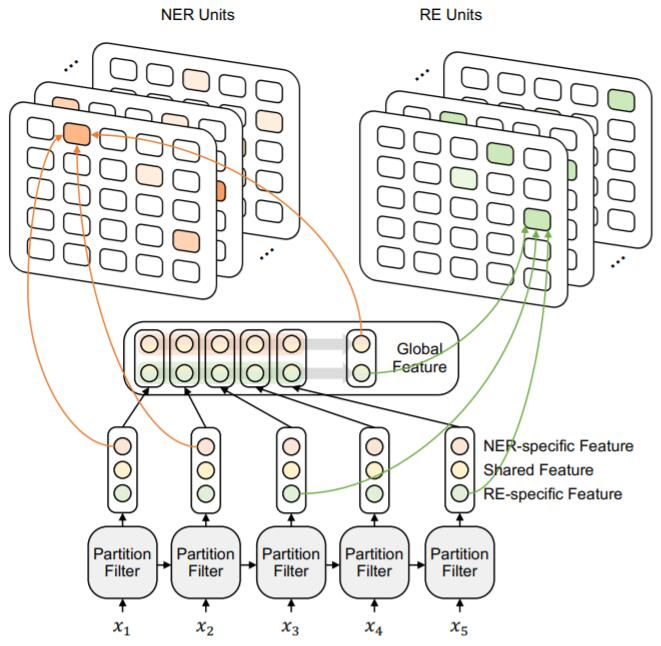## Experiments

### Main Results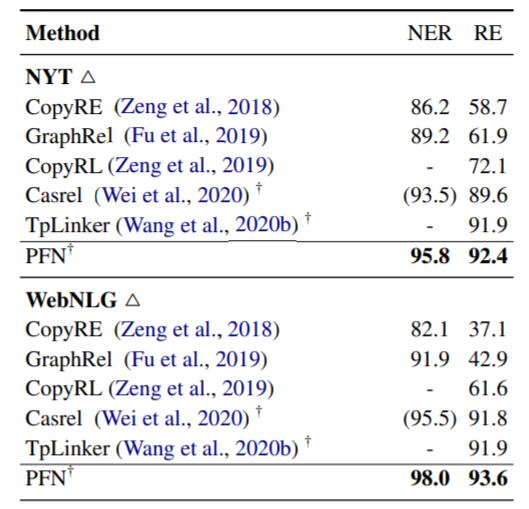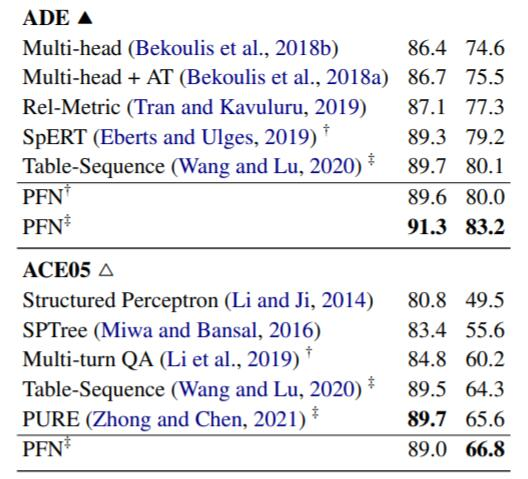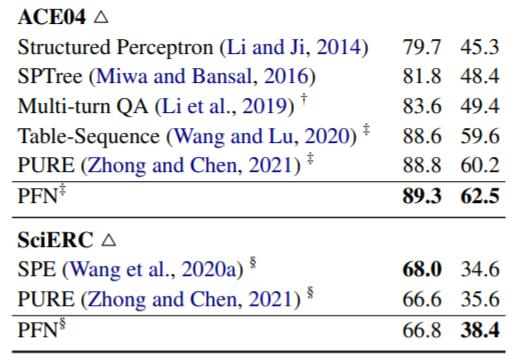PFN的性能也与强调任务间交互的Table - Sequence和Pipeline设计模型PURE(也是本系列前两篇文章介绍的模型)各有优劣, 整体上来说PFN要好. PURE在ACE05的NER上性能仍为最强.

### Ablation Study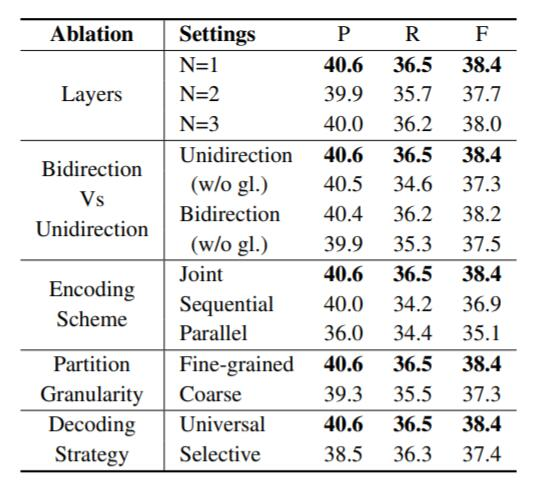#### Decoding Strategy

Selective是将NER Unit预测得出的有效实体对送给RE Unit解码的结果.

### Effects of Relation Signal on Entity Recognition

#### Analysis on Entity Prediction of Different Types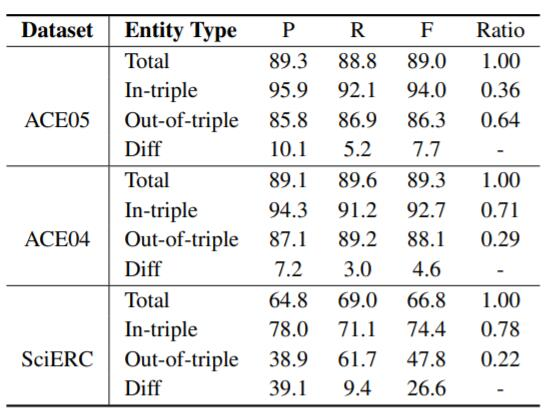#### Robustness Test on Named Entity Recognition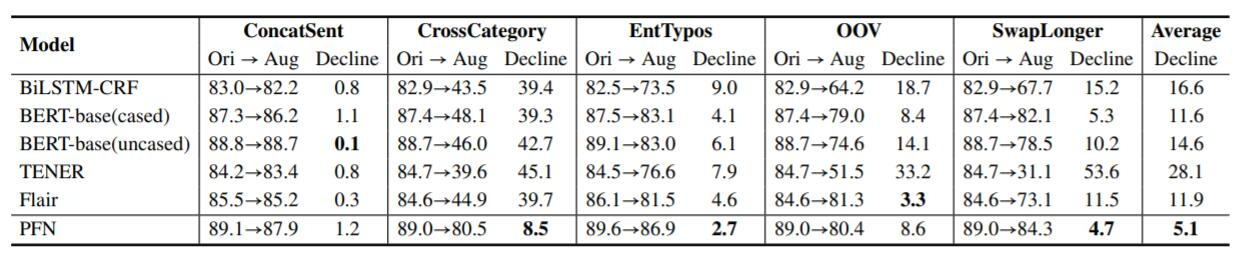#### Does Relation Signal Helps in Predicting Entities

PURE中的实验是在ACE05上做的, 而忽略了ACE05上的三元组外实体的巨大影响.

## Summary

PFN进一步的说明了联合抽取中NER和RE任务之间是密不可分的, 并有效驳斥了PURE中的论点.

上一篇SDN: Synchronous Dual Network with Cross-Type Attention for Joint Entity and Relation Extraction

2022-03-16PURE: A Frustratingly Easy Approach for Entity and Relation Extraction
A Frustratingly Easy Approach for Entity and Relation Extraction本文是论文A Frustratingly Easy Approach for Entity and Relati
2021-12-01
目录